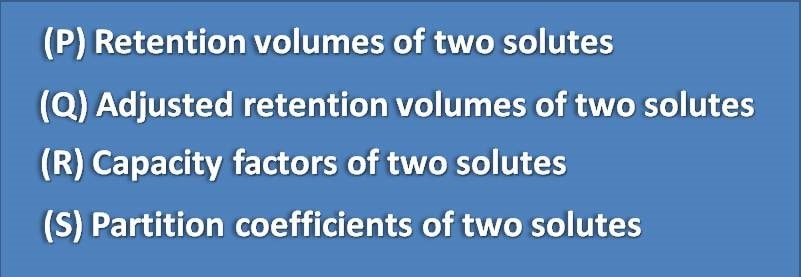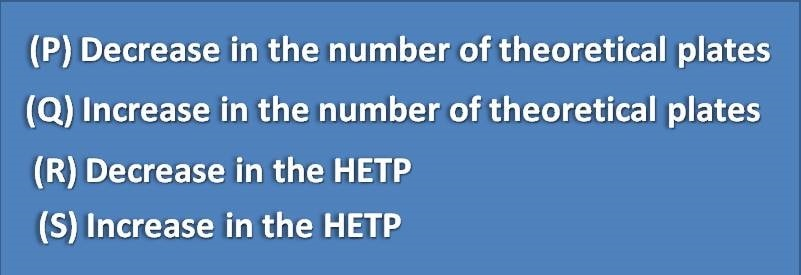1. Home
2. â€º
3. Test papers
4. â€º

# MCQ on principles of chromatography: Page-3

Selectivity factor is the ration of(A) P,S

(B) Q,R,S

(C) P,Q,R

(D) R,S

Selectivity factor for two components A & B having distribution constants K A & K B is,

(A) KB/KA

(B) K A* K B

(C) K A+K B

(D) K B-K A

The band broadening is expressed by(A) S

(B) R

(C) Q

(D) P

Which of the following is a macroporous polymer column

(A) Porous silica columns

(B) Pellicular silica columns

(C) Stryene-divinyl benzene polymer columns

(D) Porous alumina columns

The efficiency of chromatographic separation increases with(P) Decrease in the number of theoretical plates(Q) Increase in the number of theoretical plates

(R) Decrease in the HETP(S) Increase in HETP

(A) Q,S

(B) P,R

(C) Q,R

(D) P,Q